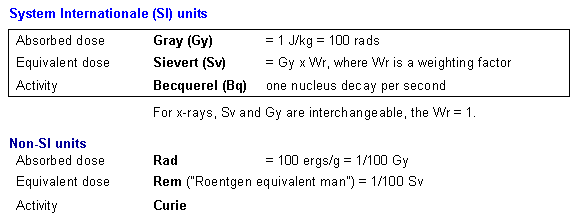##### Radiobiology > Background > Dose Terminology

Dose Units & Exposure TerminologyAbsorbed dose

• is the amount of energy transferred into a given mass.
• The SI unit is the Gray (Gy) but the older, non-SI unit, the rad, is also commonly employed.

Equivalent dose

• adjusts the absorbed dose by applying a weighting factor to account for differences among types of radiation in their capacity to cause biological damage.
• The SI unit is the Sievert (Sv)  equals Gy for x-ray energies, which are given a weighting factor of 1.

Note: Effective dose expands the weighting factor idea  weighting the equivalent dose to account for differences in radiation susceptibility among various organ systems.Note the Roentgen is the "radiation intensity" needed to produce ionization of 0.000258 coulombs per kilogram of air. Its chief use is in calibration of x-ray equipment. Also, fluoroscopic dose rates are sometimes expressed in Roentgen (R) per minute. 1 R/min = 8.7 mGy/min air kerma;

© Copyright Rector and Visitors of the University of Virginia 2021